Download Presentation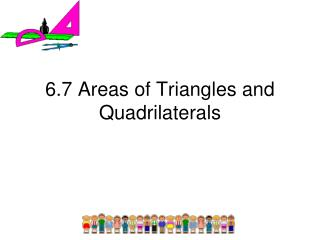6.7 Areas of Triangles and Quadrilaterals

# 6.7 Areas of Triangles and Quadrilaterals - PowerPoint PPT Presentation

6.7 Areas of Triangles and Quadrilaterals. Objectives/Assignment. Find the areas of squares, rectangles, parallelograms and triangles Find the areas of trapezoids, kites and rhombuses Assignment: 2-52 even. Using Area Formulas. Postulate 22 : Area of a SquareI am the owner, or an agent authorized to act on behalf of the owner, of the copyrighted work described.
Download Presentation## 6.7 Areas of Triangles and Quadrilaterals

An Image/Link below is provided (as is) to download presentation

Download Policy: Content on the Website is provided to you AS IS for your information and personal use and may not be sold / licensed / shared on other websites without getting consent from its author.While downloading, if for some reason you are not able to download a presentation, the publisher may have deleted the file from their server.

- - - - - - - - - - - - - - - - - - - - - - - - - - E N D - - - - - - - - - - - - - - - - - - - - - - - - - -
Presentation Transcript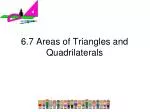### 6.7 Areas of Triangles and Quadrilaterals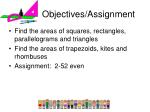Objectives/Assignment
• Find the areas of squares, rectangles, parallelograms and triangles
• Find the areas of trapezoids, kites and rhombuses
• Assignment: 2-52 even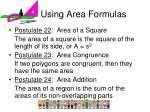Using Area Formulas
• Postulate 22: Area of a Square

The area of a square is the square of the length of its side, or A = s2

• Postulate 23: Area Congruence

If two polygons are congruent, then they have the same area

• Postulate 24: Area Addition

The area of a region is the sum of the areas of its non-overlapping parts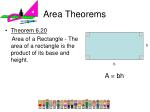Theorem 6.20

Area of a Rectangle - The area of a rectangle is the product of its base and height.

Area Theorems

h

b

A = bh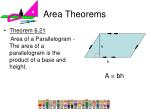Theorem 6.21

Area of a Parallelogram -The area of a parallelogram is the product of a base and height.

Area Theorems

h

b

A = bh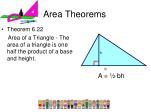Theorem 6.22

Area of a Triangle - The area of a triangle is one half the product of a base and height.

Area Theorems

h

b

A = ½ bh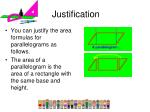The area of a parallelogram is the area of a rectangle with the same base and height.

Justification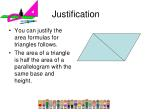You can justify the area formulas for triangles follows.

The area of a triangle is half the area of a parallelogram with the same base and height.

Justification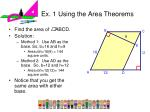Find the area of ABCD.

Solution:

Method 1: Use AB as the base. So, b=16 and h=9

Area=bh=16(9) = 144 square units.

Method 2: Use AD as the base. So, b=12 and h=12

Area=bh=12(12)= 144 square units.

Notice that you get the same area with either base.

Ex. 1 Using the Area Theorems

9

12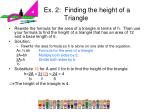Ex. 2: Finding the height of a Triangle
• Rewrite the formula for the area of a triangle in terms of h. Then use your formula to find the height of a triangle that has an area of 12 and a base length of 6.
• Solution:
• Rewrite the area formula so h is alone on one side of the equation.

A= ½ bh Formula for the area of a triangle

2A=bh Multiply both sides by 2.

2A=h Divide both sides by b.

b

• Substitute 12 for A and 6 for b to find the height of the triangle.

h=2A = 2(12) = 24 = 4

b 6 6

The height of the triangle is 4.A triangle has an area of 52 square feet and a base of 13 feet. Are all triangles with these dimensions congruent?

Solution: Using the formula from Ex. 2, the height is

h = 2(52) = 104 =8

13 13

Here are a few triangles with these dimensions:

Ex. 3: Finding the Height of a Triangle

8

8

8

8

13

13

13

13Theorem 6.23

Area of a Trapezoid - The area of a trapezoid is one half the product of the height and the sum of the bases.

A = ½ h(b1 + b2)

Areas of Trapezoids

b1

h

b2Theorem 6.24

Area of a Kite - The area of a kite is one half the product of the lengths of its diagonals.

A = ½ d1d2

Areas of Kites

d1

d2Theorem 6.25

Area of a Rhombus - The area of a rhombus is one half the product of the lengths of the diagonals.

A = ½ d1 d2

Areas of Rhombuses

d2

d1You will have to justify theorem 6.23 in Exercises 58 and 59. You may find it easier to remember the theorem this way. Areas of Trapezoids, Kites and Rhombuses

b1

h

b2

Height

Area

Length of

Midsegment

x

=Find the area of trapezoid WXYZ.

Solution: The height of WXYZ is h=5 – 1 = 4

Find the lengths of the bases.

b1 = YZ = 5 – 2 = 3

b2 = XW = 8 – 1 = 7

Ex. 4: Finding the Area of a TrapezoidEx. 4: Finding the Area of a Trapezoid

Substitute 4 for h, 3 for b1, and 7 for b2 to find the area of the trapezoid.

A = ½ h(b1 + b2) Formula for area of a trapezoid.

A = ½ (4)(3 + 7 ) Substitute

A = ½ (40) Simplify

A = 20 Simplify

The area of trapezoid WXYZ is 20 square unitsThe diagram at the right justifies the formulas for the areas of kites and rhombuses. The diagram show that the area of a kite is half the area of a rectangle whose length and width are the lengths of the diagonals of the kite. The same is true for a rhombus.Justification of Kite/Rhombuses formulasSolution—

Method 1: Use the formula for the area of a rhombus d1 = BD = 30 and d2 = AC =40

Ex. 5 Finding the area of a Rhombus

B

15

20

20

A

C

24

15

25

D

EA = ½ d1 d2

A = ½ (30)(40)

A = ½ (1200)

A = 600 square units

Method 2: Use the formula for the area of a parallelogram, b=25 and h = 24.

A = bh = 25(24) = 600 square units

Ex. 5 Finding the area of a Rhombus

B

15

20

20

A

C

24

15

25

D

EROOF Find the area of the roof. G, H, and K are trapezoids and J is a triangle. The hidden back and left sides of the roof are the same as the front and right sides.Area of J = ½ (20)(9) = 90 ft2

Area of G = ½ (15)(20+30) = 375 ft2

Area of H = ½ (15)(42+50) = 690 ft2

Area of K = ½ (12)(30+42) = 432 ft2

The roof has two congruent faces of each type

Total area=2(90+375+690+432)=3174

The total area of the roof is 3174 square feet.

SOLUTION: